Definitions of Square Dance Calls and Concepts

Index -->  Plus  |  A1  |  A2  |  C1  |  C2  |  C3A  |  C3B  |  C4  |  NOL  |
Definitions (Text Only) -->  Plus  |  A1  |  A2  |  C1  |  C2  |  C3A  |  C3B  |  C4  |  NOL  |
 Find call:

Reverse Checkpoint {anything} By {anything} [C3B]
(Vic Ceder 1985)

 \$B8@8l(B

From applicable formations. EN: 10
\$BE,@Z\$J(B formation \$B\$+\$i(B. JP: 10

Center 4 do the first anything call and the resulting Ends (of that Center 4) move away from the Center of the Set without changing their facing direction (i.e., Stable). The Others (original Outsides) work around the Very Centers to do the second anything call, ending as close as possible to the positions vacated by the resulting Ends of the Center 4. EN: 20
Center 4 \$B\$,;O\$a\$N(B anything \$B\$N%3!<%k\$r9T\$\$(B, \$B\$=\$N(B 4 \$B?M\$G(B Ends \$B\$H\$J\$C\$?(B \$B?M\$O(B, \$B8~\$-\$rJQ\$(\$:\$K(B (\$B\$9\$J\$o\$A(B Stable \$B\$G(B) Set \$B\$N(B Center \$B\$+\$iN%\$l\$^\$9(B. \$BB>\$N?M(B (\$B%*%j%8%J%k\$N30\$N?M(B) \$B\$O(B, Very Centers \$B\$N2s\$j\$G(B 2 \$B\$DL\\$N(B anything \$B\$N%3!<%k\$r9T\$\$(B, Center 4 \$B\$N(B Ends \$B\$H\$J\$C\$??M\$,6u\$1\$?>l=j\$X2DG=\$J8B\$j6a\$E\$-\$^\$9(B. JP: 20

Reverse Checkpoint Recycle By Touch 1/4: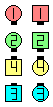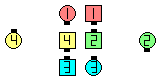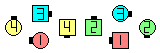Reverse CheckpointRecycle By Touch 1/4\$B\$NA0(B Centers Recycle and the resulting Ends slide away from the center EN: 30 AddJapanese \$B\$N8e(B original Outsides work aroundthe Very Centers to do a Touch 1/4 EN: 40 AddJapanese \$B\$N8e(B (\$B=*\$o\$j(B)
Reverse Checkpoint 1/4 Thru By Follow Thru: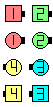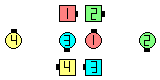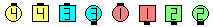Reverse Checkpoint1/4 Thru By Follow Thru\$B\$NA0(B Centers 1/4 Thru and the resulting Ends slide away from the center EN: 50 AddJapanese \$B\$N8e(B original Outsides work aroundthe Very Centers to do a Follow Thru EN: 60 AddJapanese \$B\$N8e(B (\$B=*\$o\$j(B)

Dancing Hint: The original Outsides should usually delay moving until the Centers have almost completed the first anything call, so that sufficient space is available for the Outsides to execute the second anything call. EN: 70
\$B%@%s%9\$N%R%s%H(B: \$B%*%j%8%J%k\$N30\$N?M\$O(B, \$BIaDL(B, Centers \$B\$,;O\$a\$N(B anything \$B\$N%3!<%k\$r\$[\$\=*\$(\$k\$^\$GBT\$D\$Y\$-\$G\$9(B. \$B\$=\$&\$9\$l\$P(B, \$B30B&\$N?M\$K\$H\$C\$F(B, 2 \$B\$DL\\$N(B anything \$B\$N%3!<%k\$r\$9\$k>l=j\$,==J,\$K\$G\$-\$^\$9(B. JP: 70

Note: The original Center 4 become the Very Centers and Very Outsides of the final ending formation. EN: 80
\$BCmJP: 80

Checkpoint anything By anything [C2] (Lee Kopman 1982):
From a 1 x 8, Point-to-Point Diamonds, or any other applicable formation in which there are Centers of each side. The Centers of each side work together, concentrically, around the Very Center dancers, to do the first anything call (if possible ending so that they have changed the long axis of the starting formation); Remaining Outsides (2 or 4 dancers) move into the Center (without changing their facing direction) and work with the original Centermost dancers to do the second anything call. EN: 756
1 x 8, Point-to-Point Diamonds, \$B\$^\$?\$OB>\$N\$\$\$m\$\$\$m\$JE,@Z\$J(B formation \$B\$G!V(Beach side \$B\$K(B Centers \$B\$,\$\$\$k\$H\$3\$m!W\$+\$i(B. Each side \$B\$N(B Centers \$B\$,(B, \$B\$*8_\$\$\$K0l=o\$K(B (\$BH?BPB&\$N(B Centers \$B\$H(B) \$BF0\$-(B, concentric \$B\$K(B, Very Centers \$B\$N2s\$j\$G(B, \$B;O\$a\$N(B anything \$B\$N%3!<%k\$r9T\$\$\$^\$9(B (\$B\$b\$72DG=\$J\$i(B, \$B;O\$a\$ND9<4\$KD>8r\$9\$k>l=j\$X9T\$-\$^\$9(B). \$BBT\$C\$F\$\$\$?30B&\$N(B 2 \$B?M\$^\$?\$O(B 4 \$B?M\$O(B, Center \$B\$XF0\$-(B (\$B8~\$-\$rJQ\$(\$:\$K(B), \$B%*%j%8%J%k\$N(B Very Centers \$B\$H0l=o\$K(B, 2 \$B\$DL\\$N(B anything \$B\$N%3!<%k\$r9T\$\$\$^\$9(B. JP: 756
Checkpoint anything By anything [C2]Choreography for Reverse Checkpoint anything By anythingComments? Questions? Suggestions?